# Divergent series

(diff) ← Older revision | Latest revision (diff) | Newer revision → (diff)

A series for which the sequence of partial sums does not have a finite limit. For example, the series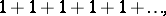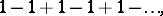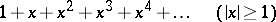are divergent.

Divergent series first appeared in the works of mathematicians of the 17th century and 18th century. L. Euler first came to the conclusion that the question must be posed, not what the sum is equal to, but how to define the sum of a divergent series, and he found an approach to the solution of this problem close to the modern one. Up till the end of the 19th century, divergent series did not find any application and were almost forgotten. Towards the end of the 19th century an accumulation of various facts in mathematical analysis re-awakened interest in divergent series. The question was raised about the possibility of summing series in a sense different from the usual one.

### Examples.

1) If one multiplies two series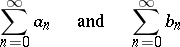that converge toand, respectively, then the resulting series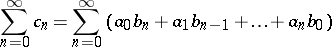(1)

can be divergent. However, if one defines the sum of the series (1) not as the limit of the partial sums, but as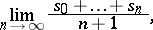(2)

then in this sense the series (1) will always converge (i.e. the limit (2) exists) and its sum in this sense is equal to.

2) The Fourier series of a functioncontinuous at a point(or having a discontinuity of the first kind) can diverge at this point. If the sum of the series is defined by formula (2), then in this sense the Fourier series of such a function will always converge and its sum in this sense will equal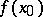(or).

3) The power series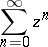(3)

converges for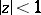to the sumand diverges for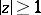. If one defines the sum of the series to be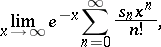(4)

where theare the partial sums of the series (3), then in this sense the series (3) will converge for allwith, and its sum is the function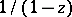.

To generalize the concept of a sum to the case of a divergent series, one takes some operator or rule which assigns a specific number to a divergent series, called its sum (in this definition). Such a rule is called a summation method (cf. Summation methods). Thus, the rule described in Example 1) is called summation by arithmetical averages (see Cesàro summation methods). The rule given in Example 2) is called the Borel summation method.